# Soil Structure Interaction (SSI)

Solution

## 1. What is Soil Structure Interaction (SSI)?

Most civil structures, especially bridges (piles, pile caps, abutments, and retaining structures) are built on or inside the ground. When analyzing such a structure, there is a great difference in the result values when the analysis is performed considering ground conditions. The ground conditions must be taken into consideration to obtain analytical results, such as the behavior of the actual structure.

•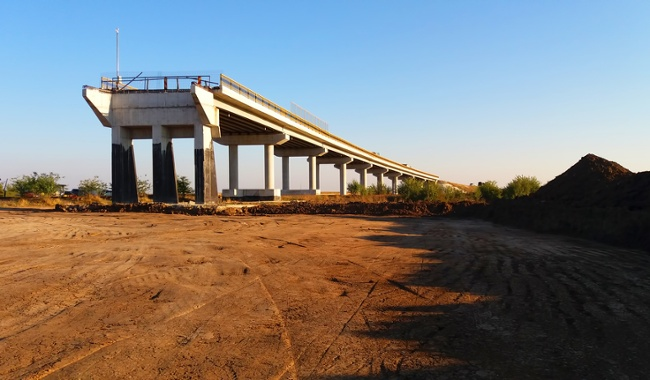Fig. Bridge structure with close relation to the ground

Soil Structure Interaction (SSI) is a physical phenomenon in which the structure fails to behave independently and behaves in connection with the soil when an external load is applied to the structure as shown in the figure below. In particular, the ground and the structures are greatly influenced by earthquakes, so this phenomenon is required as an essential consideration for seismic design.

•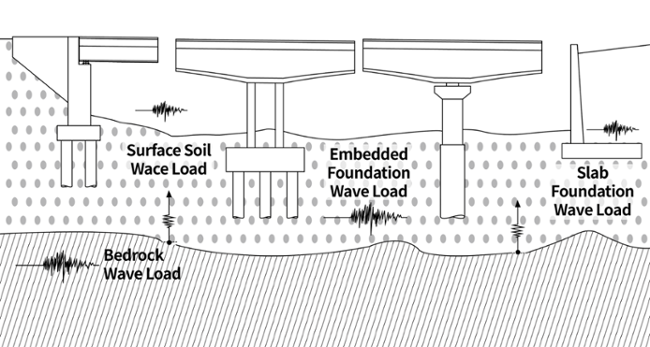Fig. Interaction between the ground and the bridge structure

Soil-structure interaction can be summarized largely in two effects. The first effect is the change in the dynamic characteristics of the structure. Because the stiffness of soil is normally smaller than that of the structure, the stiffness and the natural frequency of the structure become smaller. The second effect pertains to the increase in damping of the structural system due to the addition of radiation damping. These effects vary depending on the composition of soil layers, material properties, specified seismic data, and the frequency of the structure. Therefore, the response due to soil-structure interaction analysis can be larger or smaller than the general seismic analysis results with the assumption of fixity on the ground.

Soil response analysis is performed in order to generate design response spectrums, establish the dynamic stress-strain relationship of the soil, and to calculate the seismic loads acting on the ground structures due to an earthquake. The characteristic of the seismic wave at a bedrock outcrop is almost similar to that at bedrock. However, the characteristic of a seismic wave in soft soil or at the ground surface of the deep ground can widely vary depending on soil properties. The dynamic behavior of a structure on (or inside) soft soil can be greatly affected by soil-structure interaction. If a structure is on a bedrock where the shear strain is less than 10−6 and the velocity of the shear wave is greater than 1,100m/sec, the soil-structure interaction can be ignored.

Why do we need Soil Structure Interaction?

The need for SSI can be explained by comparing the deformation of the structure according to the difference in stiffness/strength between the structural system and the soil. In order to compare the response of the structure according to geotechnical conditions, the two conditional structures listed in the ATC-40 (Applied Technology Council) are compared.

•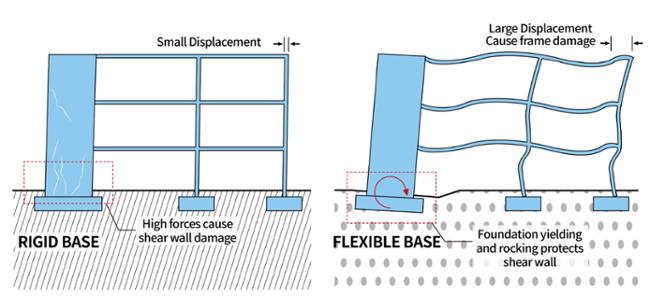Fig. Structure tesponse comparison according to ground conditions

a) For a rigid base structures, the rigidity is very high, so no additional deformations occur in the structure. Therefore, the shear and moment caused by the seismic force is only resisted by the strength of the shear wall. As a result, damage (ex. cracks) form on the shear wall, but no damages other than the small displacement occur on the frame.

b) For a flexible base, since it cannot resist deformation of structure caused by seismic forces, rotation occurs in the shear wall. This rotation induces deformation to the frame, and as a result causes large deformations and cracks to the frame structure. However, the force demand of the shear wall decreases compared to a).

It can be seen that the analysis results for both cases are extremely different depending on the soil conditions. If the ground condition is not properly considered and interpreted as a rigid base as shown in a), the shear wall will be overdesigned and the frame will not be properly designed. The response of the structure as in case b) can be evaluated as the difference between the ground motion without the structure and the motion of the structure due to the stiffness of the foundation, and also as an additional displacement in the ground caused by the force induced by the motion of the structure. These mechanical phenomena are referred to as Kinematic Interaction and inertial interaction, respectively.

In SSI analysis, the linear/nonlinear behavior of the structure and the ground, as well as the nonlinear behavior at the contact surface between the structure and the ground (sliding, rocking) should be considered. Analytical methods can be largely divided into Direct and Substructure methods, depending on numerical modeling methods or seismic input methods.

•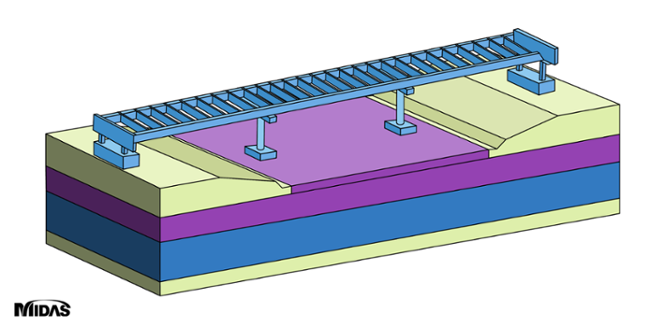Fig. Soil Structure Interaction analysis method (direct method)

•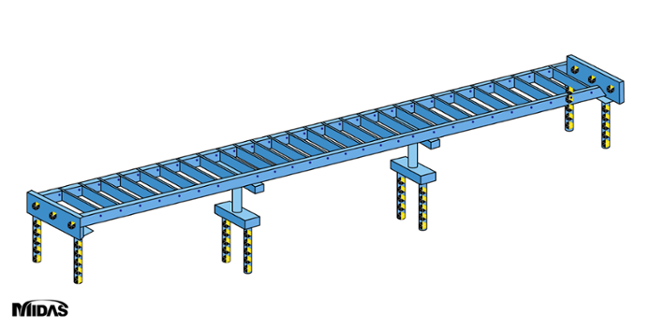Fig. Soil Structure Interaction analysis method (substructure method)

## 2. Mechanical Classification of Soil Structure Interaction

The effects of SSI can be classified into Kinematic Interaction and Inertia Interaction. Kinematic Interaction is due to the stiffness difference between the soil and the structure, and Inertia Interaction is due to the inertia force of the structure.

### A. Kinematic Interaction

When a seismic force is applied, a difference occurs between the ‘Free Field Ground Motion’ (absence of any structure) and the ‘Foundation Input Motion’

(absence of mass in the upper structure). This difference is referred to as Kinematic interaction and includes a combination of geometry of structures, rigidity difference between foundations and soils, embedment effects and base-Slab averaging effect. Furthermore, this action can be quantified by the Transfer function as the ratio between the ‘Free Field Ground Motion’ and the ‘Foundation Input Motion’.

Since the incident wave with a high order frequency component retains a short wavelength, the stiffness of the foundation absorbs and reflects the wave. However, an incident wave with a low order frequency component retains a long wavelength, which passes through the structure and generates motion in the structure. In case of a vertically incident shear wave, rocking motion is generated in the structure. The ground motion generated by kinematic interaction is also referred to as scattering motion. Kinematic interaction is generally affected by the type of incident wave and the shape of the foundation. If the structure is on the ground surface, the foundation is embedded into the ground at a shallow depth. On the other hand, if the surface wave is not considered, the effect of kinematic interaction is not so significant that it may be ignored during seismic analysis.

•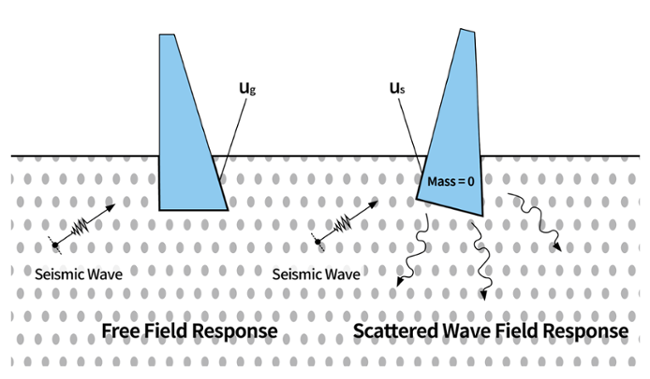Fig. Free-Field response and scattered wave field response

##### Free Field Motion

Free-Field is referred to as the location where the structure is absent, and the design ground motion is defined as ground motion in the free-field. The point where the design ground motion is defined is called the control point, and the ground motion at that location is called the control motion.
ASCE 4, Design ground motions are defined as the free-field response (acceleration, velocity, displacement) that is the site response in the absence of structure.

##### Base-Slab Averaging Effect

Effect of free-field response across contact surfaces at the foundation of the structure being constrained by the stiffness of the base slab.

•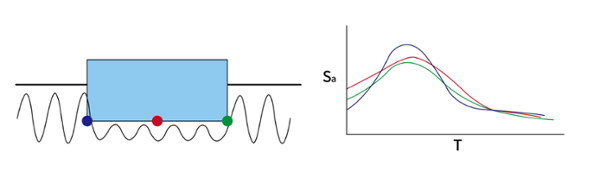Fig. Base slab averaging effect

##### Embedment Effect

The effect of decreasing response along the depth of the structure.

•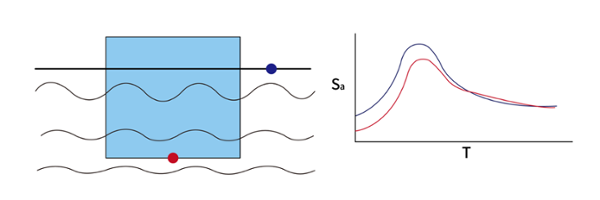Fig. Embedment effect

### B. Inertia Interaction

Inertia Interaction refers to a phenomenon in which the inertial force of a structure caused by seismic forces causes relative displacement between the ground and the structure, resulting in Hysteretic Soil Damping and Radiation Damping.

•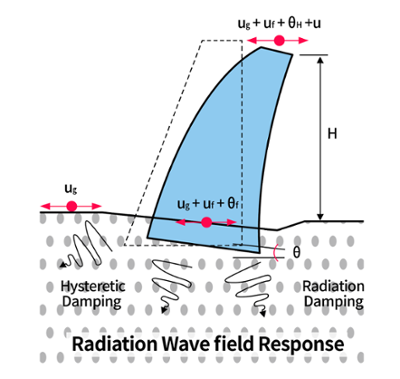Fig. Response dictated by interaction between soil structure

When a horizontal seismic force is applied to the base, the final displacement generated on the structure includes sliding due to the horizontal movement, and rocking due to rotation. This displacement occurs from the shear force and moment created on the foundation base by seismic forces. These displacements, which cause deformation on the ground, are referred to as inertia interaction and quantified by the Impedance function.

When the structure is excited by seismic waves, the inertia force is generated by the structure’s mass. The inertia force in turn generates kinematic energy which is then radiated to the far field soil, resulting in radiation motion of the ground. Inertial interaction increases as the stiffness difference between the structure and the nearby ground increases. Therefore, when a very stiff structure is situated in soft soils, the influence of inertia interaction is much greater.

##### Hysteretic Damping

A phenomenon caused by material properties, in which kinetic energy and deformation energy are converted into heat due to friction between soil particles resulting in loss of energy

A phenomenon in which the energy of a structure is dissipated by releasing wave energy into the semi-infinite soil medium.

## 3. Soil Structure Interaction Analysis Method

The accuracy of the SSI analysis results depends on how radiation conditions are considered due to the infinite domain of the soil, the modeling method of soil materials, and the nonlinear modeling method between the soil and the structure. SSI can be divided mainly into two different methods. The first method is the Direct Method, which considers the ground and the structure as a complete system and models it like a structure using finite elements or finite differential methods. The second method is the Substructure Method, which treats the mechanical behavior of the soil as a single system with independent stiffness and damping.

### A. Direct Method

The Direct method is a method of directly modeling structures and soils and inputting seismic forces to the outer boundary of the analytical model. Since the Direct method models the soil, it has the advantage of taking into account soil materials and the nonlinearity between the soil and the structure. The following points should be considered when creating the analytical model. The outer boundary shall be set to an area where the stress waves generated by the structure cannot be reached. Appropriate boundary conditions must be set for the inner and outer boundaries of the analytical model, and the mesh size of the soil model must be sufficiently small. The size of the mesh is determined by considering the type of finite element, the shear wave velocity of the soil, and the maximum excitation frequency.

•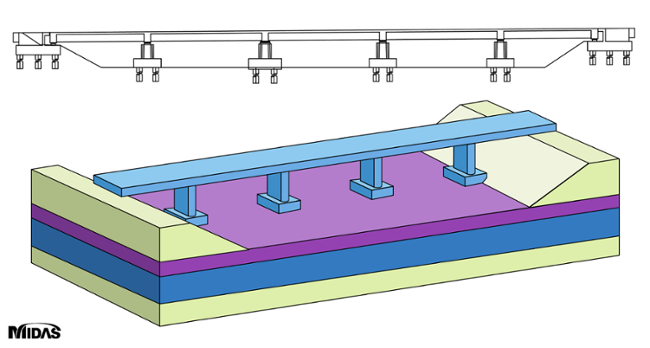Fig. Direct method modeling for SSI analysis

### B. Substructure Method

The Substructure method (or Indirect method) is the method of separating the soil and the structure and analyzing each as a separate structural system. The design seismic input given to the designer in this method is the ground motion in the Free-field. In this method, the non-correlation between the structure and the ground can be calculated through the Time history method or the response spectrum method. The calculated result is applied to the Foundation Input Motion of the Kinematic Interaction System of the SSI analysis to obtain the response of the structure considering inertial interaction.

Because of the frequency-dependence of the dynamic impedance matrix, the analysis is carried out iteratively

##### Iteration Process for Dynamic Impedance Matrix

Step1: From the controlled motion in the free-field, determine the input motion at the soil-structure interface considering the soil properties and the effect of foundations.

Step2: Define the dynamic stiffness of the semi-infinite ground at the soil-structure interface.

Step3: Model a soil-structure system by combining the dynamic soil stiffness calculated from Step2 with the structure. Thereafter, calculate the structural response by applying the excitation of the input motion obtained from Step1.

•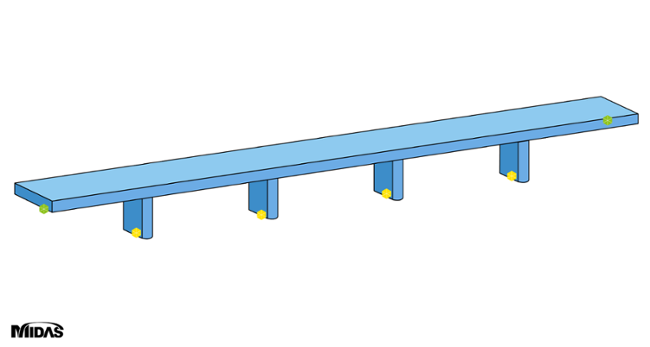Fig. Structural modeling using six degrees of freedom spring